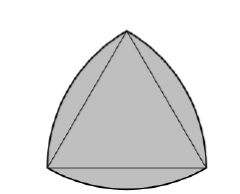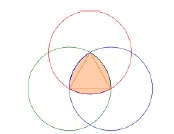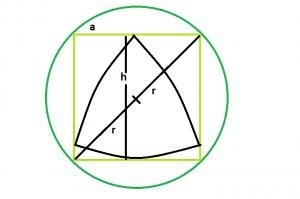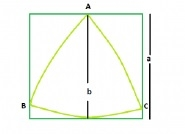# Biggest Reuleaux Triangle within a Square which is inscribed within a Circle in C?

CServer Side ProgrammingProgramming

#### C in Depth: The Complete C Programming Guide for Beginners

45 Lectures 4.5 hours

#### Practical C++: Learn C++ Basics Step by Step

Most Popular

50 Lectures 4.5 hours

#### Master C and Embedded C Programming- Learn as you go

66 Lectures 5.5 hours

Reuleaux triangle is a shape formed from the intersection of three circular disks, each having its center on the boundary of the other two. Its boundary is a curve of constant width, the simplest and best known such curve other than the circle itself. Constant width means that the separation of every two parallel supporting lines is the same, independent of their orientation. Because all its diameters are the same.The boundary of a Reuleaux triangle is a constant width curve based on an equilateral triangle. All points on a side are equidistant from the opposite vertex.To construct a Reuleaux triangle

Formula for Reuleaux triangle

Area of the Reuleaux Triangle, if curve based on an equilateral triangle and side of tringle is h

A = (π * h2) / 2 – 2 * (Area of equilateral triangle) = (π – √3) * h2 / 2 = 0.70477 * h2

Biggest Reuleaux Triangle within a Square which is inscribed within a CircleFig1.Biggest Reuleaux Triangle within a Square which is inscribed within a CircleBiggest Reuleaux Triangle within A Square

Area of Reuleaux Traingle is 0.70477 * b2 where b is the distance between the parallel lines supporting the Reuleaux Triangle.

distance between parallel lines supporting the Reuleaux Triangle = Side of the square i.e. a

Area of the Reuleaux Triangle, A = 0.70477 * a2

Let’s take an example to illustrate the concept better,

Input: r = 6
Output: 50.7434

## Explanation

The side of the square is a, then

a√2 = 2r

a = √2r

in reuleaux triangle, h = a = √2r,

Area of the Reuleaux Triangle is, A = 0.70477*h^2 = 0.70477*2*r^2

## Example

#include <stdio.h>
#include<math.h>
int main() {
float r = 6;
float area = 0.70477 * 2 * pow(r, 2);
printf("The area is : %f",area);
return 0;
}

## Output

The area is : 50.743439